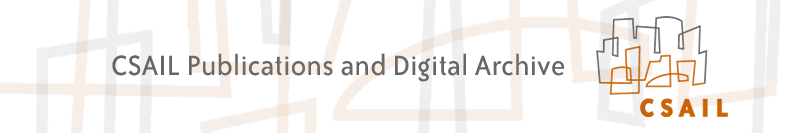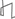Research Abstracts Home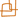CSAIL Digital Archive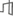Research Activities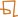CSAIL HomeResearch Abstracts - 2007IntroductionPerception & LearningPhysical, Biological & Social SystemsSystemsTheory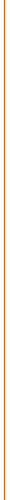### Breaking The Epsilon-Soundness Bound of the Linearity Test Over GF(2)

#### Tali Kaufman, S. Litsyn & Ning Xie

##### Background

In various papers the following problem was addressed: Given a function that is epsilon-far (e-far) from the set of linear functions, what is the lower bound on the rejection probability of the linearity test of Blum, Luby and Rubinfeld ? We denote this lower bound by Rej(e). Part of the interest in studying Rej(e) is due to its relation to PCP constructions and hardness of approximating some NP-hard problems.

##### Previous Results

The previous best bounds for Rej(e) were obtained by Bellare, Coppersmith, Hastad, Kiwi and Sudan , who showed that Rej(e) >= e for every 0 < e < 1/2. For years it seemed that breaking this epsilon-bound is a very hard problem. The bound of  is based on Fourier analysis of functions over the complex plane. The bound for Rej(e) they obtained would be tight if the range of the functions to be tested were complex numbers. However, the functions considered in the linearity test are boolean functions.

##### Our Results

One may ask if there exists a method that exploits the property of these functions being boolean to obtain a better bound. In  we give an affirmative answer to this question. Specifically, we improve the lower bound of Rej(e) by an additive constant that depends only on epsilon. Our analysis is based on a new relationship between Rej(e) and the weight distribution of a coset of the Hadamard code. Our result may serve as an explicit example showing that the Fourier analysis, though being a powerful tool, is incapable of proving optimal bounds for boolean functions.

##### Our Techniques

We use both Fourier analysis and coding theory tools to study the weight distribution of a coset of the Hadamard code for obtaining the improved bound.

##### References:

 M. Bellare, D. Coppersmith, J. Hastad, M. Kiwi and M. Sudan. Linearity testing over characteristic two. In IEEE Transactions on Information Theory, volume 42, number 6, pp. 1781--1795, 1996.

 T. Kaufman, S. Litsyn and N. Xie. Breaking The Epsilon-Soundness Bound of the Linearity Test Over GF(2). Manuscript, 2006.

 M. Blum, M. Luby and R. Rubinfeld. Self-Testing/Correcting with Applications to Numerical Problems. In Journal of Computer and System Sciences, volume 47, number 3, pp. 549--595, 1993.Computer Science and Artificial Intelligence Laboratory (CSAIL) The Stata Center, Building 32 - 32 Vassar Street - Cambridge, MA 02139 - USA tel:+1-617-253-0073 - publications@csail.mit.edu